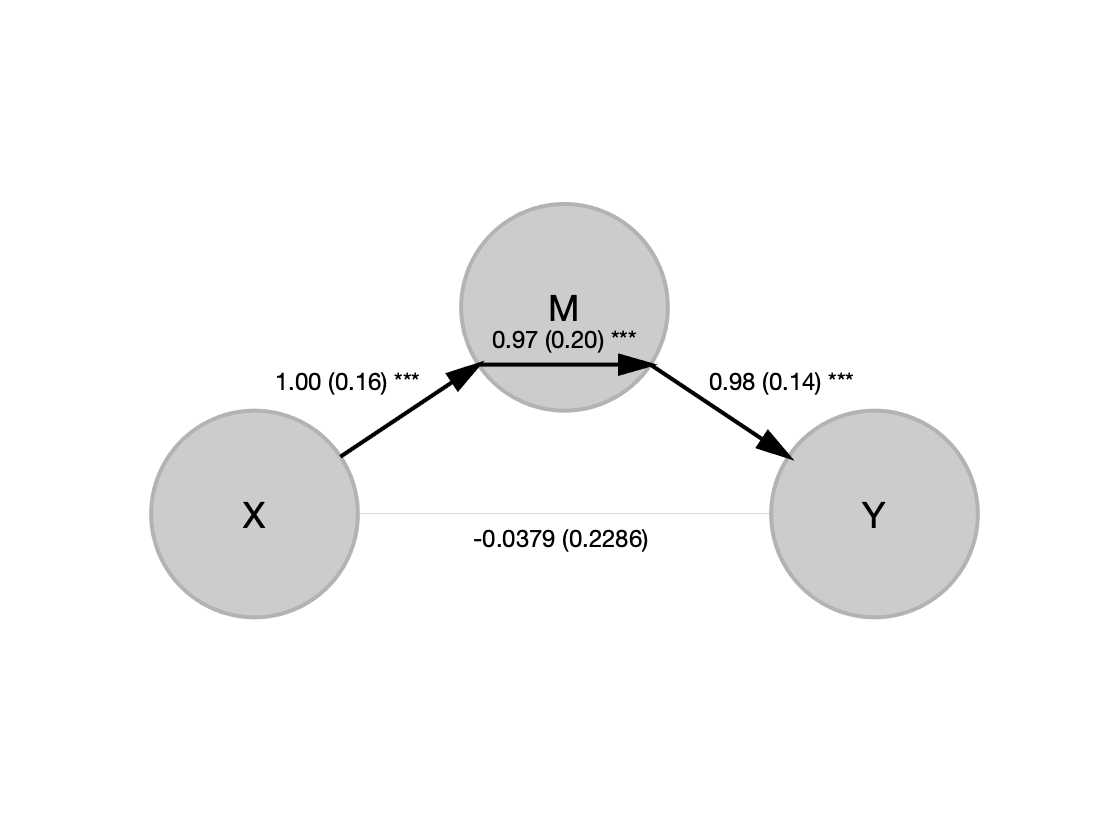# Mediation analysis: The basics

## Mediation basics

In many systems -- whether biological, mechanical, social, or information systems -- the relationship between two variables x and y may be transmitted through a third intervening variable, or mediator, m. For example, the effects of a psychological stressor (x) on heart rate (y) may be mediated by activity in brain systems responsible for threat (m). The stressor activates the brain, and the brain in turn controls the heart.
There are many other examples. Exposure to a drug may cause a clinical benefit via its effects on brain neurotransmitter levels. Solar energy may power an electric motor via an intermediate transformation to energy by a solar cell. Changing the position of an advertisement on a web page may influence sales of the advertised product via the position's intermediate effects on people's attention to the ad.
In all these cases, testing whether some of the total effect of the exposure on the outcome is transmitted through the mediator can help explain why the relationship between the variables exists and under what conditions it is likely to be found.
Mediation is a useful tool for connecting variables into pathways, . It extends the idea of a simple association between two variables (let's call them x and y) to a pathway involving three (or more) variables. Let's write the association x predicts y like this: [ x -> y ]. This relationship is shown graphically in the diagram below: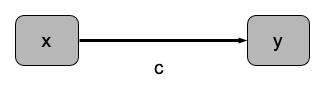Suppose we hypothesize that the effect of x on y is transmitted via its influence on an intermediate variable, m. With mediation analysis, you can build a model of associations among three variables, i.e., [ x -> m -> y ]. m is a mediator, a variable that explains some or all of the relationship between x and y.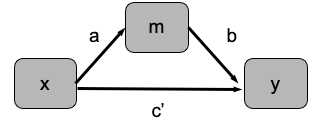Mediation models are especially useful for testing whether an association between two variables is transmitted via a third variable. For example, the effects of a psychological stressor (x) on heart rate (y) may be mediated by activity in brain systems responsible for threat. The stressor activates the brain, and the brain in turn controls the heart.
In mediation analysis, x is called the initial variable. it will often be an experimental variable (IV) that is experimentally manipulated, but it doesn't have to be. y is called the outcome variable (or dependent variable), and it is usually the endpoint that one is interested in explaining. m is the mediator, and is usually a variable that is thought to be important for transmitting the effect of x on y, or on the "causal path" from x to y. It is also possible to include multiple initial variables, multiple mediators, and other covariates in such models. The broader family that the [ x -> m -> y ] model belongs to is called path models.
Here are some other examples. Exercise may improve memory via neurogenesis. Exercise causes new neurons to be generated in the brain (neurogenesis), which helps the brain form new memories more efficiently. here, x is exercise, m is neurogenesis, and y is memory performance. Smoking (x) may lead to premature death (y) via increased rates of cancer (m).

### A graphical example of mediation

Let's create a sample dataset that would show complete mediation, where the relationship between x and y (exercise and memory performance in the previous example) is fully explained by m (neurogenesis). The generative model underlying the dataset is that m is caused by x and other unmeasured random variation (noise), and y is caused by m and unmeasured random variation.
x = [ones(25, 1); -ones(25, 1)];
m = x + randn(50, 1);
y = m + randn(50, 1);
figure; hold on;
plot(m, y, 'ko'); refline
h = []; % store handles
h(1) = plot(m(x == 1), y(x == 1), 'bo', 'MarkerFaceColor', [.2 .4 1], 'MarkerSize', 8);
h(2) = plot(m(x == -1), y(x == -1), 'go', 'MarkerFaceColor', [.4 1 .2], 'MarkerSize', 8);
xlabel('Neurogenesis (Mediator)'); ylabel('Performance (Outcome)');
legend(h, {'Exercise HIGH' 'Exercise LOW'});
set(gca, 'FontSize', 18)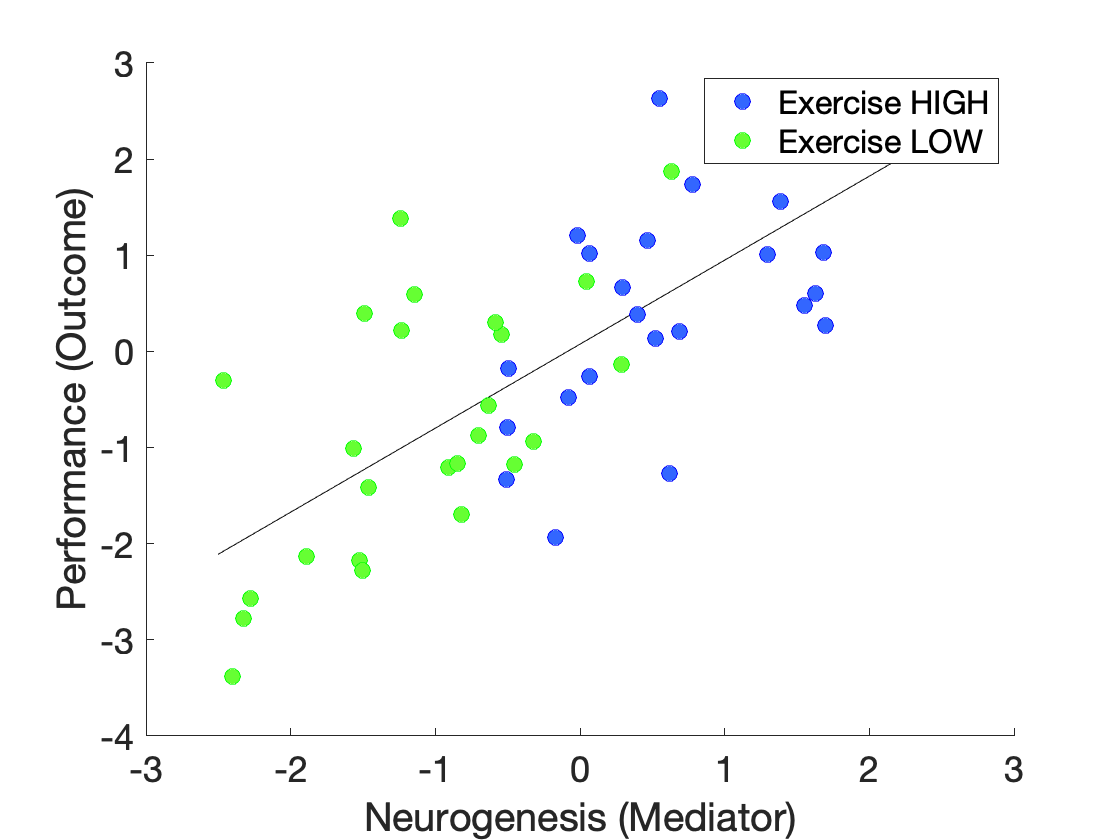Here, the Path a effect (exercise on neurogenesis) is captured by the shift over on neurogenesis (x-axis) for blue vs. green points (high vs. low exercise). The Path b effect is captured by the relationship between neurogenesis and performance (y-axis). The Path c effect (total effect) is captured in the shift up on performance for blue vs. green points. The Path a*b (mediation) effect is reflected in the fact that the shift upwards in performance (y) with exercise (x) is exactly what one would expect from the effects of exercise on neurogenesis (m). The high vs. low exercise points fall on a continuous line.
The Path c' (direct) effect is not shown here, but reflects the difference in intercept for two parallel slope lines constrained to have the same slope for high and low exercise. If the effects of exercise on performance were too large relative to the effects of neurogenesis, the blue points would lie above the green ones, and the effect of exercise would not be well explained by a linear effect of exercise.
This plot helps illustrate that a dataset may show a significant Path a and Path b effect, but no significant mediation (a*b) effect. The test of the product requires both effects to be large enough; if either is zero, for example, the product will be zero too. Conversely, the set of statistical tests that show a significant mediation effect will usually be a subset of those that show a significant Path a and Path b effect.

### Estimating path models and statistical inference

Testing for mediation involves fitting two linear regression equations. The slopes (coefficients) of these equations are called paths, and the estimated regression coefficients are path coefficients.
There are three path coefficients in the model above. The path coefficient a captures the effect of x on m (e.g., smoking on cancer in the example above). This is estimated by the first equation. The path coefficient b captures the effect of m on y (e.g., cancer on mortality in the example above), and the coefficient c’ the direct effect of x on y after controlling for m. The path through m is called the indirect pathway, and the mediation via M the indirect effect. Statistical inference on this pathway is made by testing the product of the a and b path coefficients.
The equations are: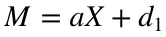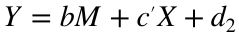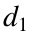and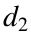are intercept (constant) terms. To test mediation, we estimate both of these equations, calculate the a*b product, and turn this into an inferential statistic (e.g., a t statistic) by dividing by an estimate of its standard error. The Sobel test is one way of doing this using a formula, but it is only asymptotically accurate in large samples and depends strongly on assumptions of normality. In practice, it is typically overconservative. Mediation is more commonly tested using bootstrapping, as described in Shrout and Bolger (2002).
Here is an example of a bootstrapped distribution for the indirect effect from the M3 Mediation Toolbox. This distribution provides confidence intervals and P-values for the a*b effect and other path coefficients: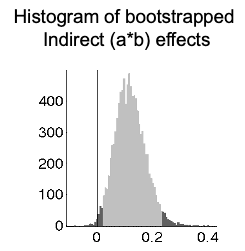The dark shading shows the top and bottom 2.5% of the distribution, and thus the 95% confidence interval for a*b. If 0 falls outside of this (in the dark gray zone), the effect is significant at P < 0.05.
In whole-brain mediation analysis, we bootstrap path coefficients and the mediation effect at each brain voxel, and save maps of P-values. These are subjected to voxel-wise False Discovery Rate correction to correct for multiple comparisons.

### Counterfactual interpretation of mediation

A way of understanding the mediation effect is in terms of a counterfactual: What x -> y association would we have observed if we had prevented m from varying?
We can't directly observe the counterfactual case, but we can test the difference between the simple x -> y effect when we don't control for the mediator (Path c in the figure above) with the x -> y effect when we do control for the mediator (Path c'). Thus, we want to statistically test whether c - c' = 0.
It turns out that with a little algebraic manipulation, we can show that: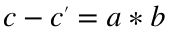So a statistical test of a*b is the same as comparing the effect of x on y across two models: One in which the mediator can vary, and one in which it's statistically adjusted for -- the regression equivalent of holding it constant.

### Mediation vs. moderation

Moderators are variables that interact with the initial variable to affect the outcome. This is conceptually distinct from a mediator, although variables in some physical systems can serve as both mediators and moderators. Causal mediation analysis also considers these effects jointly.
With moderators, the level of the moderator affects the relationship between x and y. For example, neurogenesis may promote memory for historical facts, but only because it potentiates the effects of studying those facts. In this case, studying would be a moderator (Mo) of the effects of neurogenesis (x) on memory performance (y).
This is typically tested by adding an interaction term to the regression model. This amounts to a statistical test of the difference in the slope of the [x -> y] relationship when the moderator is high vs. low. We create an interaction variable by multiplying the scores for X and Mo for each observation, and including this as a regressor.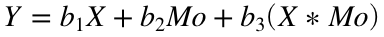Here, the moderation effect is captured by slope parameter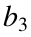. We'd typically include the other main effects (of X and Mo) in the regression model as well. With interactions, the scaling and centering of the variables also matters. It's typical to mean-center the variables X and Mo before creating the interaction regressor. That way, the interaction is orthogonal to the two main effects.
Moderation is often shown graphically by a line from the moderator variable to the arrow connecting x and y. Here is an example, from Wager, Scott, & Zubieta 2007. The relationship between opioid binding in the anterior cingulate and PAG is moderated by the presence of a placebo treatment that induces expectation and, apparently, opioid release common to these interconnected brain regions. Each dot is one person, and the moderation (interaction) effect tests whether the slopes differ for placebo On vs. Off.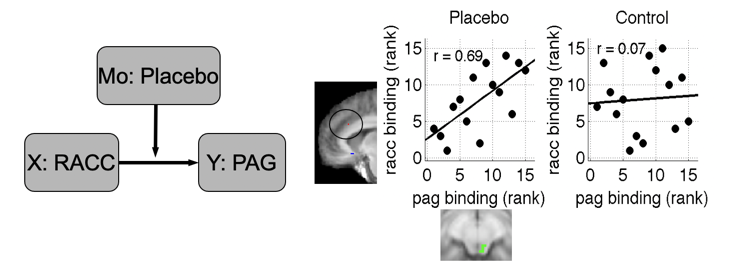Moderation is statistically distinct from mediation, although a variable can be both a mediator and a moderator. For example, exercise may increase neurogenesis, which may mediate effects on cognitive performance. Neurogenesis is a mediator, but it can also be a moderator, if the effect of exercise on performance is stronger when neurogenesis is higher.

### Multilevel mediation

In the standard mediation model, each of X, M, and Y are vectors with one observation per person. Thus, the individual participant is the unit of observation. When this is the case, we'll call this a single-level mediation. 99% or so of the mediation analyses in the literature are single-level models. For example, X can include a vector of individuals who are either smokers (coded as 1) or non-smokers (coded as -1), and we include measures of both the incidence of cancer (M) and survival time (Y) for each person.
What happens if we have repeated observations of X, Y, and M within a person? This allows us to estimate the Path a, Path b, and a*b effects within each person. This allows each person "to serve as their own control", and eliminates a number of person-level confounds and noise. For example, in our cancer example above, people will likely vary in their diet, and diet could be correlated with smoking, introducing a potential confound (or "3rd variable") and at the least an additional source of uncontrolled noise. In a mulitlevel model, we can estimate the effect within-person, and test the significance of the path coefficients across individuals, treating participant as a random effect. This reduces the chances that the mediation effect is related to a 3rd variable, and allows us to generalize the effect across a population with a range of diets (and other person-level characteristics). This can often dramatically increase the power and validity of a mediation model.
In addition, with multilevel mediation, we can include person-level covariates in the model as well. For example, we can test whether diet moderates (influences) the effects of smoking on cancer (Path a), the effects of cancer on mortality (Path b), or the mediation (a*b) effect. This is called moderated mediation. Thus, multilevel mediation allows us to include two kinds of variables in the same model: within-person variables that are measured for each person, and between-person (or person-level) variables that are measured once for a person. We can make inferences on the within-person mediation effect and test whether it generalizes over or is moderated by person-level characteristics.
Single-level (standard) and multilevel mediation with all of these variations is implemented in the Multilevel Mediation and Moderation (M3) Matlab Toolbox.

### A note on causality

Mediation models of the type we explore here (and path models more generally) are build on linear regression, with the same assumptions and limitations of other linear models. It is a directional model: As with a standard multiple regression model, we are explaining y as a function of x and m. And, as with standard regression, the presence of a statistical association does not provide direct information on causality. The observed relationships could, in principle, be caused by other variables that are not included in the model (i.e., lurking variables or common causes), and/or the direction of causality can be reversed from how you've formulated it in the model.
Experimental manipulation of variables can provide stronger evidence for causal associations, and sometimes both x and m are experimentally manipulated. This is a major strength of experimental methods across all disciplines! However, when variables are observed rather than manipulated, statistical associations very rarely provide strong information about causality. In particular, when x and m are both observed (not manipulated), reversing the mediation (i.e., swapping x and m) is not a good way to determine what the form of the model should be. This is because differences in relative error (or reliability) could make one model fit better than another, irrespective of the true causality.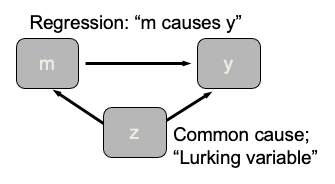An alternative to the linear model approach we describe here is the causal mediation framework, which makes the assumptions required for causal inference more explicit, and provides tests for mediation based on conditional probabilty (essentially, stratification). One entry point for this is Robins and Greenland (1992) and Pearl (2014). However, there is no "magic bullet" for assuring causality if the assumptions underlying causal inference might not be valid. It's worth taking a closer look at these here. To infer causality, we need more than just the variables we're measuring: We need solid information about the broader system in which the variables are embedded.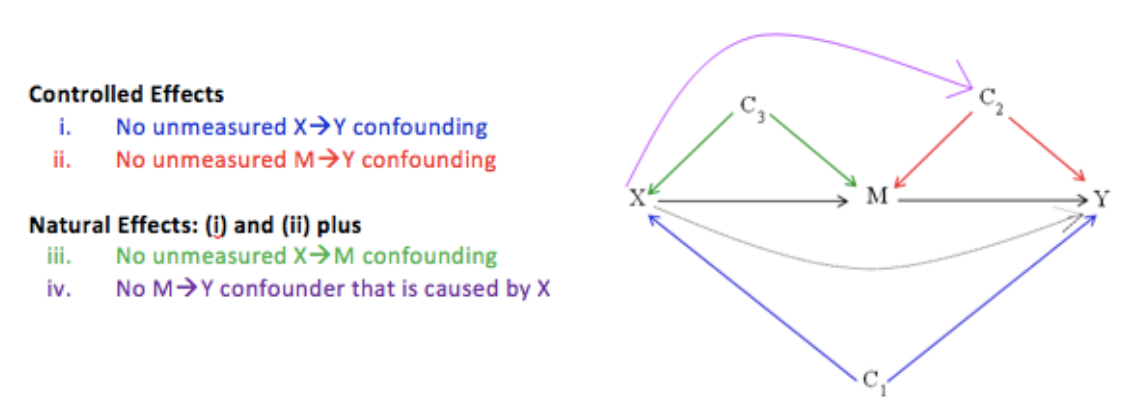The image shown below is from the Columbia causal mediation website.

There is a huge literature on mediation analysis, as it's been used in tens of thousands of papers across fields. More details on basic principles of mediation, with references, can be found on David Kenny's excellent web page, and in a number of papers and books (e.g., MacKinnon 2008).

## The M3 Mediation Toolbox

The multilevel mediation and moderation (M3) toolbox is a Matlab toolbox designed for mediation analysis. It permits tests of both single-level and multi-level mediation. It was developed in part for use with neuroimaging data, but can be used for any type of data, including behavior/performance, survey data, physiological data, and more.

### Features

The M3 toolbox provides several features tailored for mediation analysis. These are available when analyzing any kind of data, and are also available when performing voxel-wise analyses of neuroimaging data:
• Single or multi-level mediation
• Bias corrected, accelerated bootstrapping for inference
• Precision-weighted estimates for mixed effects (multi-level)
• Logistic regression for categorical outcomes (Y)
• Ability to add multiple mediators and covariates
• Second-level moderators for moderated mediation
• Multi-path (3-path) mediation
• Permutation testing for inference
• Latent hemodyamic response basis set and time-shift for fMRI mediators
• Autocorrelation estimation (AR(p)) for time series mediators
• Multivariate mediation to identify a pattern across dense, high-dimensional mediators

### Installation and dependencies

See the README.md file on the Mediation Toolbox Github site.

### Main functions to run

The function mediation.m is the main function to run from the Matlab command line for a standard mediation analysis.
The toolbox also has special functions for Mediation Effect Parametric Mapping, the practice of running mediation on each voxel in a neuroimaging dataset (single or multilevel) and saving maps of mediation effects:
mediation_brain % for single-level mediation
mediation_brain_multilevel % for multilevel mediation
The toolbox also has the ability to perform Multivariate mediation to identify a pattern across dense, high-dimensional mediators. See the bibliography below, and other tutorials in this series, for examples of voxel-wise mediation effect mapping and multivariate mediation.

### Acknowledgements and key references

Acknowledgements
This toolbox was developed with the generous support of the U.S. National Science Foundation (NSF 0631637, Multilevel mediation techniques for fMRI) to Tor Wager and Martin Lindquist. We are grateful to Prof. Niall Bolger and Prof. Michael Sobel for helpful discussions and input.
References
The key papers describing the toolbox are below. It would be helpful to cite these papers when using the M3 toolbox. The manuscripts and supplementary information contain fairly complete descriptions of the model and statistical procedures used.
Shrout PE, Bolger N (2002) Mediation in experimental and nonexperimental studies: New procedures and recommendations. Psychol Methods 7:422–445.
A classic reference for bootstrap-based inference in mediation.
Kenny DA, Korchmaros JD, Bolger N (2003) Lower level mediation in multilevel models. Psychol Methods 8:115–128.
A seminal paper on multi-level mediation.
Wager, T. D., Davidson, M. L., Hughes, B. L., Lindquist, M. A., & Ochsner, K. N. (2008). Prefrontal-subcortical pathways mediating successful emotion regulation. Neuron, 59(6), 1037-1050. doi:10.1016/j.neuron.2008.09.006
This paper describes Mediation Effect Parametric Mapping with bootstrap-based inference and examples using multiple mediators. It describes and provides the first application of the canonical case where M is a set of brain images, and X and Y are given. It also describes suppressor effects and search when the initial variable (X) is the brain variable, and M and Y are given.
Wager, T. D., Waugh, C. E., Lindquist, M., Noll, D. C., Fredrickson, B. L., & Taylor, S. F. (2009). Brain mediators of cardiovascular responses to social threat, Part I: Reciprocal dorsal and ventral sub-regions of the medial prefrontal cortex and heart-rate reactivity. NeuroImage, 47, 821-835.
This paper describes multilevel mediation as applied to fMRI time series data, and provides the first application of multilevel mediation to fMRI data.
Wager, T. D., van Ast, V. A., Hughes, B. L., Davidson, M. L., Lindquist, M. A., & Ochsner, K. N. (2009). Brain mediators of cardiovascular responses to social threat, Part II: Prefrontal-subcortical pathways and relationship with anxiety. NeuroImage, 47, 836-851.
This paper describes multi-level mediation on fMRI time series, including the first application of moderated mediation and analyses of time-lagged mediators (mediators for which the time constants in relation to physiological outcomes differ across brain regions).
Atlas, L. Y., Bolger, N., Lindquist, M. A., & Wager, T. D. (2010). Brain mediators of predictive cue effects on perceived pain. J Neurosci, 30(39), 12964-12977. doi:10.1523/JNEUROSCI.0057-10.2010
This paper applies multi-level mediation to single-trial brain images in fMRI, connecting an experimental design variable (X), brain mediators (M), and a behavioral outcome (Y), including second-level moderators. It provides the first application of multilevel mediation to single-trial fMRI data, and includes an extensive supplementary information document with more details on multilevel mediation.
Woo, C. W., Roy, M., Buhle, J. T., & Wager, T. D. (2015). Distinct brain systems mediate the effects of nociceptive input and self-regulation on pain. PLoS biology, 13(1), e1002036. doi:10.1371/journal.pbio.1002036
This paper applies multi-level mediation to single-trial fMRI data, and provides the first application of multi-path (3 path) mediation, developed by Choong-Wan Woo.
Oliver Y Chén, Ciprian Crainiceanu, Elizabeth L Ogburn, Brian S Caffo, Tor D Wager, Martin A Lindquist, High-dimensional multivariate mediation with application to neuroimaging data, Biostatistics, Volume 19, Issue 2, April 2018, Pages 121–136.
This paper describes the statistical foundations of multivariate mediation, and is the primary statistical reference for this technique.
Stephan Geuter, Elizabeth A Reynolds Losin, Mathieu Roy, Lauren Y Atlas, Liane Schmidt, Anjali Krishnan, Leonie Koban, Tor D Wager, Martin A Lindquist, Multiple Brain Networks Mediating Stimulus–Pain Relationships in Humans, Cerebral Cortex, Volume 30, Issue 7, July 2020, Pages 4204–4219
This paper describes the application of multivariate mediation to a large single-trial fMRI dataset, and illustrates how mediation can be combined with other tools to interpret high-dimensional patterns of brain mediators.

## Basic single-level mediation analysis using the M3 toolbox

Let's try a basic analysis using the toolbox. You'll need the mediation toolbox installed and on your Matlab path, along with the CANlab Core toolbox. If you type
which mediation
/Users/torwager/Documents/GitHub/MediationToolbox/mediation_toolbox/mediation.m
...and you get an error, it's not installed correctly.

### Create simulated data

First, we'll create some simulated data. The initial variable, X, has 50 observations (e.g., participants). M is a noisy copy of X, and Y is a noisy copy of M. There is no direct effect (X -> Y independent of M), so M should be a complete mediator, with a non-significant path c', but a significant a, b, and a * b mediation effect.
X = rand(50, 1);
M = X + rand(50, 1);
Y = M + rand(50, 1);
whos X Y M
Name Size Bytes Class Attributes M 50x1 400 double X 50x1 400 double Y 50x1 400 double

### Estimate the model

Next, we'll estimate the mediation model using mediation.m
[paths, stats] = mediation(X, Y, M, 'plots', 'verbose');
Mediation analysis Observations: 50, Replications: 1 Predictor (X): X, Outcome (Y): Y: Mediator (M): M Covariates: No Single-level analysis. Options: Plots: Yes Bootstrap: No Robust: No Logistic(Y): No Done.
Mediation scatterplots: Replications: 1 Covariates controlled for in all regressions: 0 predictors Additional mediators controlled for in outcome predictions: 1 predictors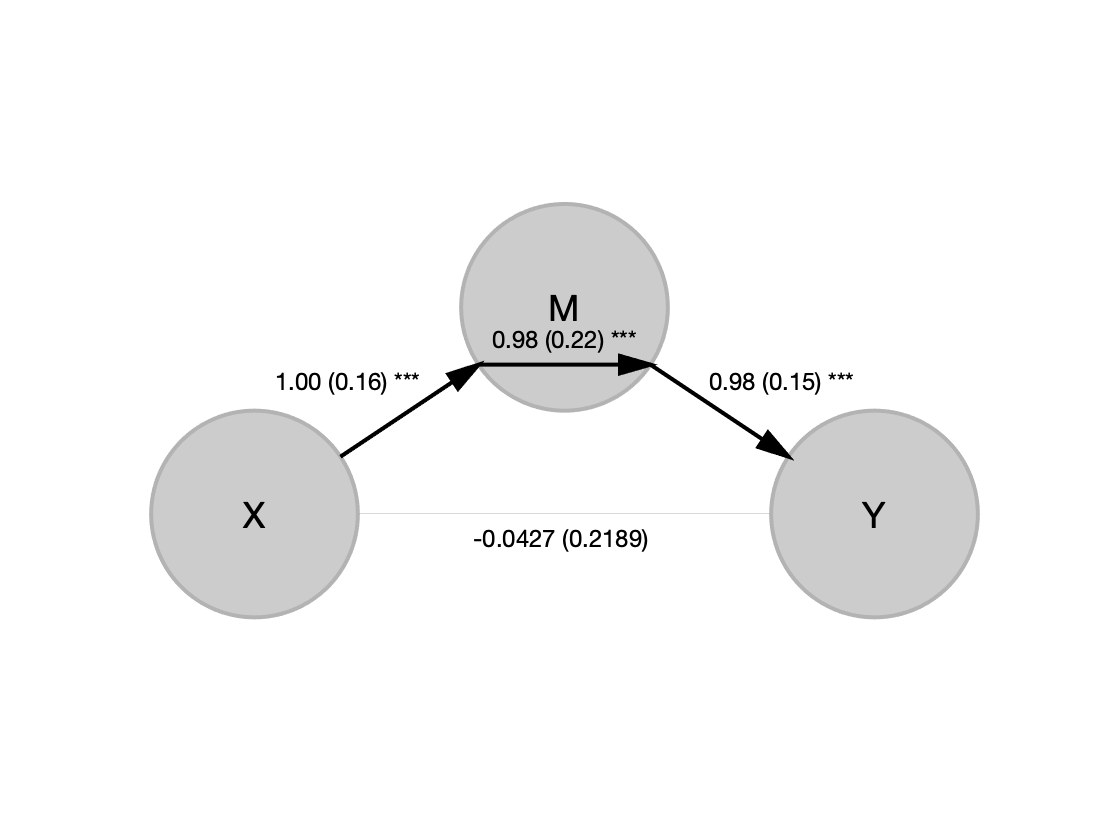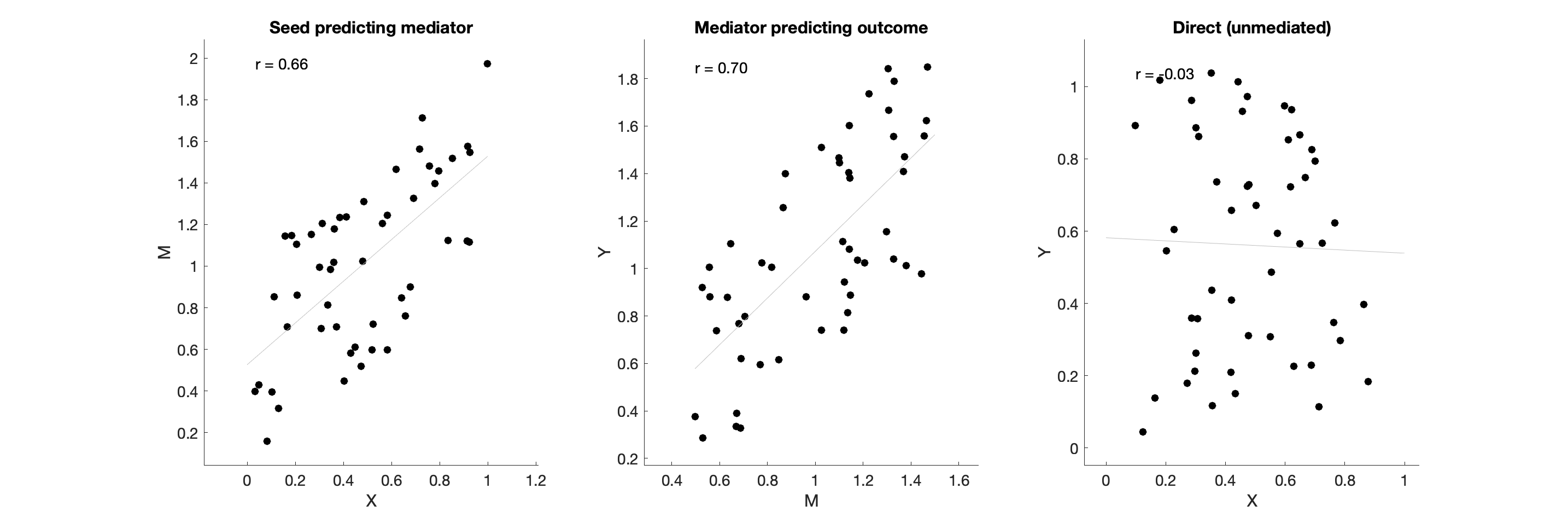________________________________________ Single-level model a b c' c ab Coeff 1.00 0.98 -0.04 0.94 0.98 STE 0.16 0.15 0.22 0.23 0.22 t (~N) 6.14 6.78 -0.20 4.13 4.53 Z 5.26 5.65 -0.19 3.81 4.11 p 0.0000 0.0000 0.8461 0.0001 0.0000 ________________________________________ Total time: 2 s
In the output, Replications: 1 means that this is a single-level analysis. We have not replicated within-person mediation effects in multiple individuals. Each observation (row of X, Y, or M) is a single score from a single participant.
The path diagram shows significant Path a (X -> M) and Path b (M -> Y) effects at P < 0.001 (***). The unstandardized path coefficients are shown, with their standard errors in parentheses. The direct effect (X -> Y) is shown by a light gray line because it is not signfiicant after controlling for M.
Note: Your results may vary due to chance if you run this, but you should get something very close to the above on average.
The scatterplots show the partial regression plots. The table shows the path coefficients (unstandardized) for each effect. Paths a, b, and c' are as above. Path c is the total effect (X -> Y, not controlling for the mediator). Path ab is the mediation effect, or indirect effect.

#### Output variables

Several variables are returned to the workspace. These are:
paths a vector of path coefficients a, b, c', c, and ab
stats a structure with lots of info, including paths, statistics (t and P-values), residuals, and more.
stats contains the information used to generate the output table when you enter the 'verbose' keyword.

### Bootstrapping and other common options

Note that we didn't use bootstrap tests here, because this isn't used by default. Let's use the 'boot' input keyword to turn that on:
[paths, stats] = mediation(X, Y, M, 'boot', 'verbose');
Mediation analysis Observations: 50, Replications: 1 Predictor (X): X, Outcome (Y): Y: Mediator (M): M Covariates: No Single-level analysis. Options: Plots: No Bootstrap: Yes Robust: No Logistic(Y): No Bootstrap or sign perm samples: 1000 Bootstrapping: Min p-value is 0.001000. Adding 4867 samples Adding 4867 samples Done adding in 0 s Done in 0 (s) Done. ________________________________________ Final bootstrap samples: 5867 Average p-value tolerance (average max alpha): 0.0060 Single-level model a b c' c ab Coeff 1.00 0.98 -0.04 0.94 0.97 STE 0.16 0.14 0.23 0.24 0.20 t (~N) 6.26 6.99 -0.16 3.98 4.93 Z 3.74 3.52 -0.20 3.36 3.63 p 0.0002 0.0004 0.8424 0.0008 0.0003 ________________________________________ Total time: 0 s
Now we get bootstrapped paths and stats. mediation.m has estimated how many bootstrap samples we need given our input number (1000 by default if we do not enter a number) and a comparison of the minimum P-value and our desired precision. For example, the min P-value the toolbox will return with 1,000 bootstrap samples is 0.001 (1/1000). If we want to be able to estimate stronger effects with lower P-values, we need more bootstrap samples. This isn't an issue here, but could be if we wanted to correct for mulitple comparisons across many mediation tests. Here it's added 5,000 or so additional bootstrap samples so that we can get more precise (and lower) P-values when the effects are strong.
We can also choose a high number of bootstrap samples, which is great for moderate-size datasets because they run fast:
[paths, stats] = mediation(X, Y, M, 'boot', 'verbose', 'bootsamples', 10000);
Mediation analysis Observations: 50, Replications: 1 Predictor (X): X, Outcome (Y): Y: Mediator (M): M Covariates: No Single-level analysis. Options: Plots: No Bootstrap: Yes Robust: No Logistic(Y): No Bootstrap or sign perm samples: 10000 Bootstrapping: Min p-value is 0.000100. Adding 0 samples Done in 0 (s) Done. ________________________________________ Single-level model a b c' c ab Coeff 1.00 0.98 -0.04 0.94 0.97 STE 0.16 0.14 0.23 0.24 0.20 t (~N) 6.29 6.84 -0.17 3.97 4.87 Z 3.89 3.63 -0.23 3.68 3.76 p 0.0001 0.0003 0.8208 0.0002 0.0002 ________________________________________ Total time: 0 s
We haven't asked for plots, so we don't get any...but we can pass the stats structure to other functions in the toolbox to get those:
mediation_path_diagram(stats)
mediation_scatterplots(stats)
Mediation scatterplots: Replications: 1 Covariates controlled for in all regressions: 0 predictors Additional mediators controlled for in outcome predictions: 1 predictors